# Create a custom number format in excel

Feb 04, 2020 • edited Feb 05, 2020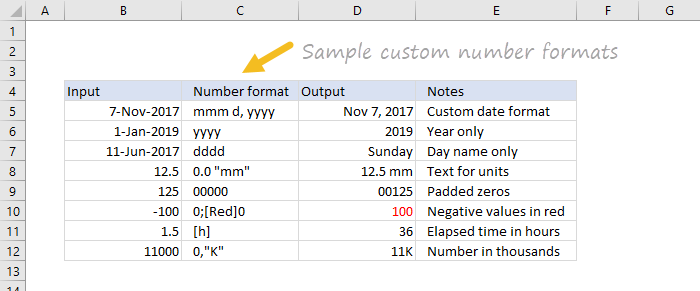# Custom Number Format

Number formats control how numbers are displayed in Excel. The key benefit of number formats is that they change how a number looks without changing any data. They are a great way to save time in Excel because they perform a huge amount of formatting automatically.

If a built-in number format does not meet your needs, you can create a new number format that is based on an existing number format and add it to the list of custom number formats. For example, if you're creating a spreadsheet that contains customer information, you can create a number format for telephone numbers.

learn how to create a custom number format in Excel to display dates in different ways, display special characters (like arrows) and change the color to red for negative numbers.

Excel has many built-in formats that you can use: Currency, Accounting, Date, Time, Percentage, etc. If you cannot find the right format, you can create a custom number format.

For example, you might have codes that consist of 5 numbers. Instead of typing 00041, simply type 41 and let Excel add the leading zeros.

1. Enter the value 41 into cell A1.

2. Select cell A1, right click, and then click Format Cells.

3. Select Custom.

4. Type the following number format code: 00000

5. Click OK.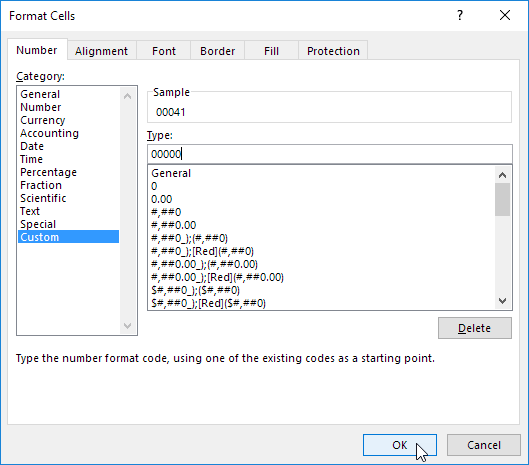Note: Excel gives you a life preview of how the number will be formatted (under Sample).

Result: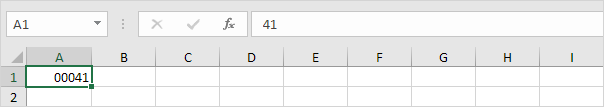Note: cell A1 still contains the number 41. We only changed the appearance of this number, not the number itself.

### Decimal Places

You can also control the number of decimal places. Use 0 to display the nearest integer value. Use 0.0 for one decimal place. Use 0.00 for two decimal places, etc.

1. Enter the value 839.1274 into cell A1.

2. Use the following number format code: 0.00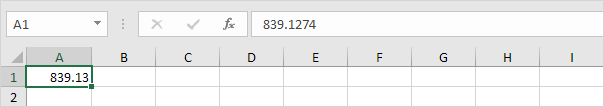1. Enter the value 839.1274 into cell A1.

2. Use the following number format code: 0.0 “ft”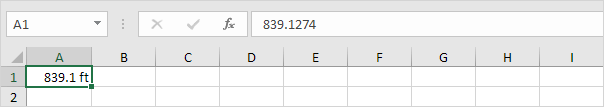Note: remember, we only changed the appearance of this number, not the number itself. You can still use this number in your calculations.

### Large Numbers

You can also control large numbers. Use one comma (,) to display thousands and use two commas (,,) to display millions.

1. Enter the following values in cells A1, B1, C1 and D1: 1000000, 2500000, 81000000 and 700000.

2. Use the following number format code: 0.0,, “M”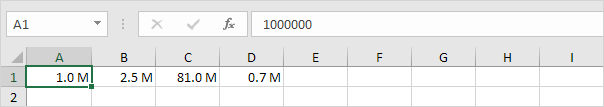Note: we used 0.0 for one decimal place and “M” to add the letter M.

### Repeat Characters

Use the asterisk (*) followed with a character to fill a cell with that character.

1. Type Hi into cell A1.

2. Use the following number format code: *@ -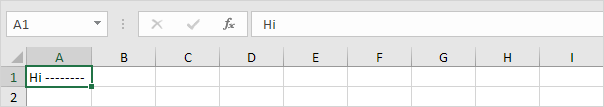Note: the @ symbol is used to get the text input.

### Colors

You can control positive numbers, negative numbers, zero values and text all at the same time! Each part is separated with a semicolon (;) in your number format code.

1. Enter the following values in cells A1, B1, C1 and A2: 5000000, 0, Hi and -5.89.

2. Use the following number format code: **[Green]\$#,##0_);[Red]\$(#,##0);“zero”;[Blue]“Text:” @**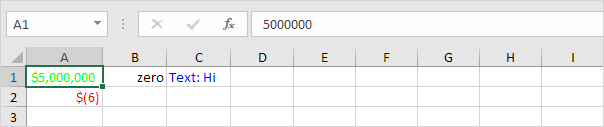Note: #,## is used to add comma's to large numbers. To add a space, use the underscore “_” followed by a character. The length of the space will be the length of this character. In our example, we added a parentheses “)". As a result, the positive number lines up correctly with the negative number enclosed in parentheses. Use two parts separated with a semicolon (;) to control positive and negative numbers only. Use three parts separated with a semicolon (;) to control positive numbers, negative numbers and zero values only.

### Dates and Times

You can also control dates and times. Use one of the existing Date or Time formats as a starting point.

1. Enter the value 42855 into cell A1.

2. Select cell A1, right click, and then click Format Cells.

3. Select Date and select the Long Date.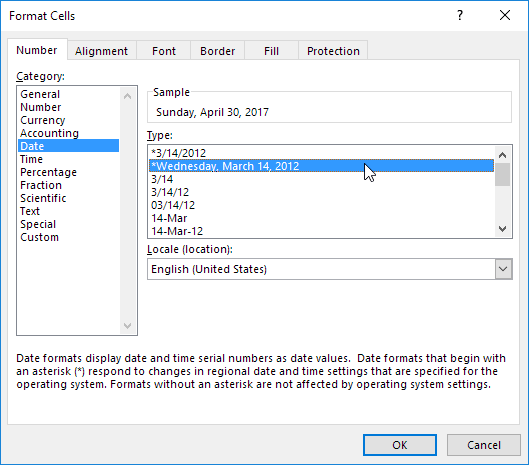Note: Excel gives you a life preview of how the number will be formatted (under Sample).

4. Select Custom.

5. Slightly change the number format code to: mm/dd/yyyy, dddd

6. Click OK.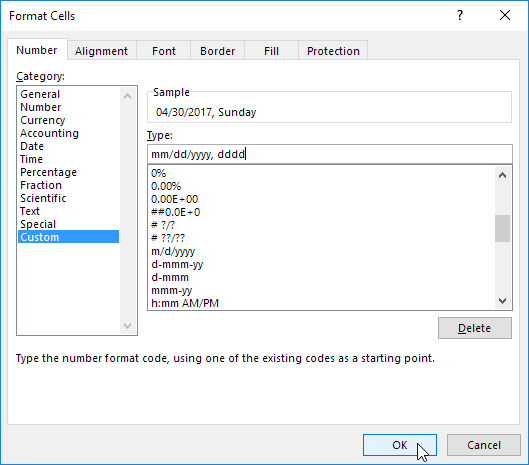Result: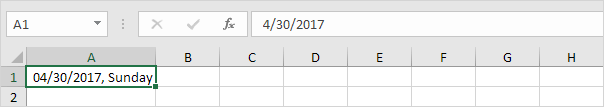General note: a custom number format is stored in the workbook where you create it. If you copy a value with a custom number format to another workbook, it will also be available in that workbook.

#How To#Tutorial#Format Cells

How to Use Format Painter to Copy Formatting in Excel

How to convert numbers to text in excel